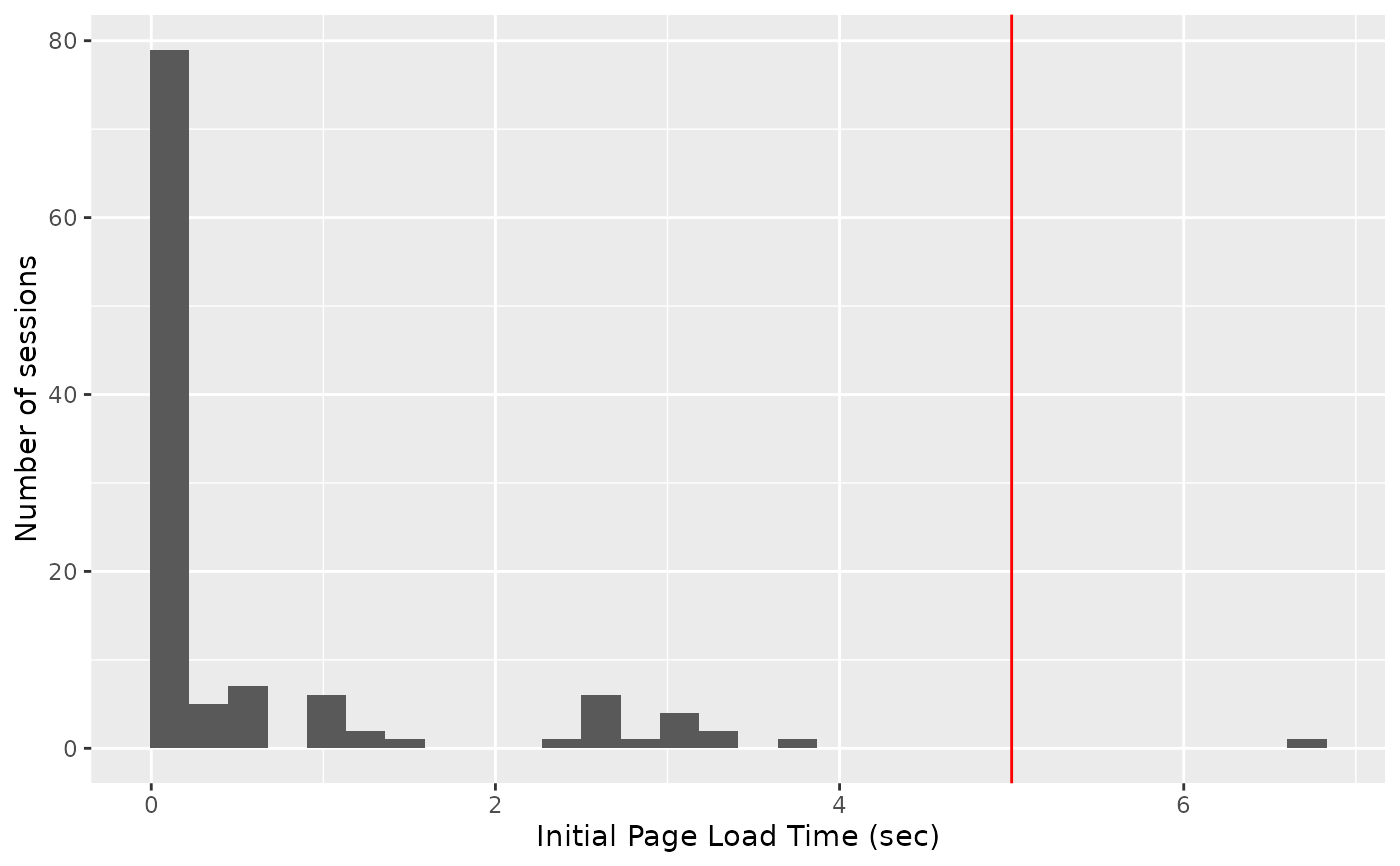Many different plotting routines to display different loadtest information.

slt_time_boxplot(df, labels = NULL)

slt_time_concurrency(df, labels = NULL)

slt_waterfall(df, limits = NULL)

slt_user(df)

slt_session(df)

slt_session_duration(df, cutoff = NULL)

slt_session_latency(df)

slt_http_latency(df, cutoff = 5)

slt_websocket_latency(df, cutoff = 5)

## Arguments

df

data frame returned from load_runs

labels

A vector of labels to include. If none are supplied, all labels will be used.

limits

passed into scale_colour_gradientn

The amount of time users will wait for the page to load when first requesting the app.

cutoff

Where to draw a horizontal or vertical line to display a reasonable cutoff line for requests.

## Value

A ggplot plot object

## Functions

• slt_time_boxplot: Box plot of load times for each event in each run

• slt_time_concurrency: Time on concurrency for each event for each run

• slt_waterfall: Event waterfall for each session within each run

• slt_hist_loadtimes: Histogram of page load times

• slt_user: Gantt chart of event duration for each user within each run

• slt_session: Event gantt chart of each user session within each run

• slt_session_duration: Event gantt chart of fastest to slowest session times within each run

• slt_session_latency: Stacked bar chart of event duration for each session within each run

• slt_http_latency: Bar chart of total HTTP latency for each session within each run

• slt_websocket_latency: Bar chart of maximum calculation (websocket) latency for each session within each run

## Examples

slt_user(slt_demo_data_4)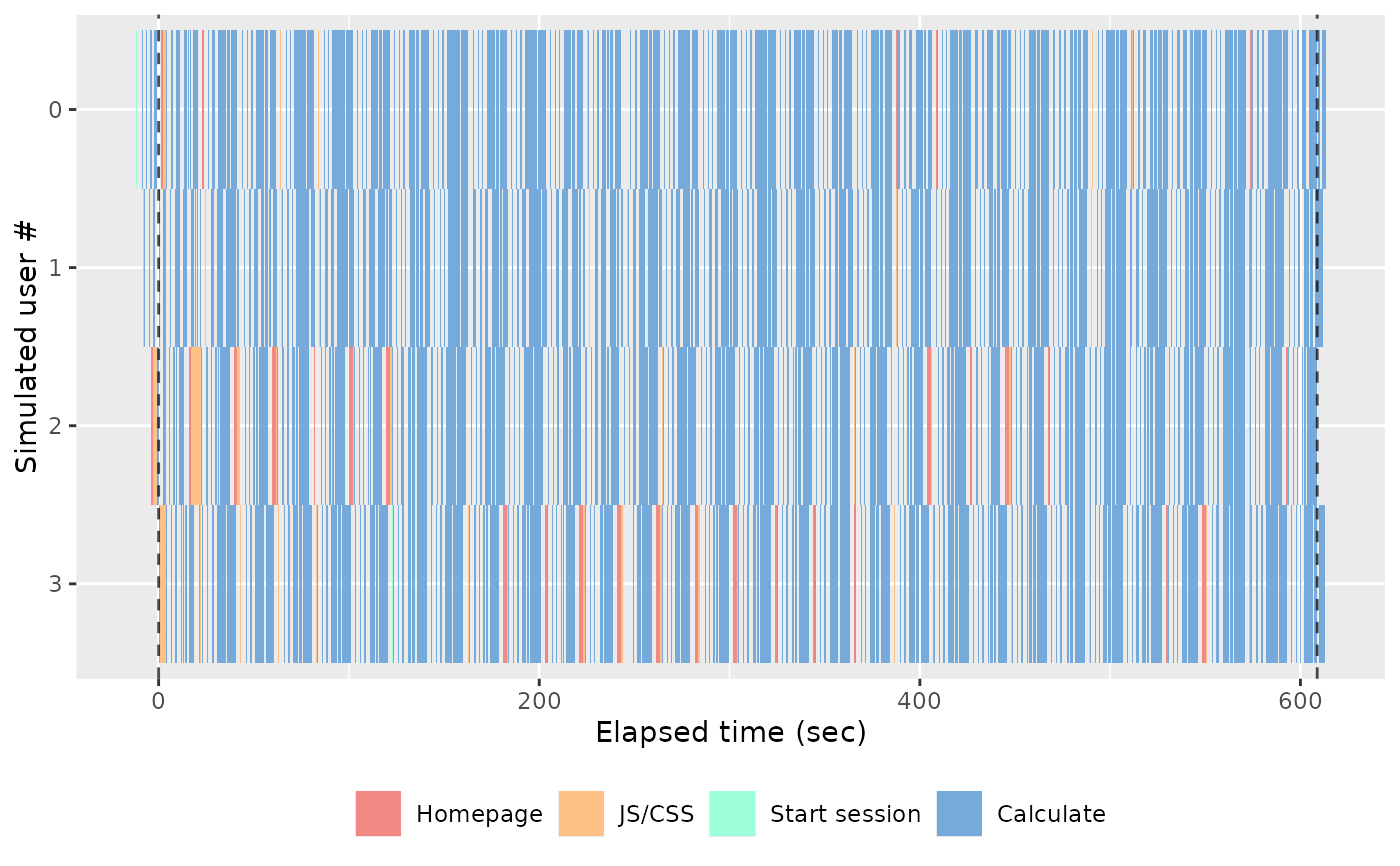slt_session(slt_demo_data_4)slt_session_duration(slt_demo_data_4)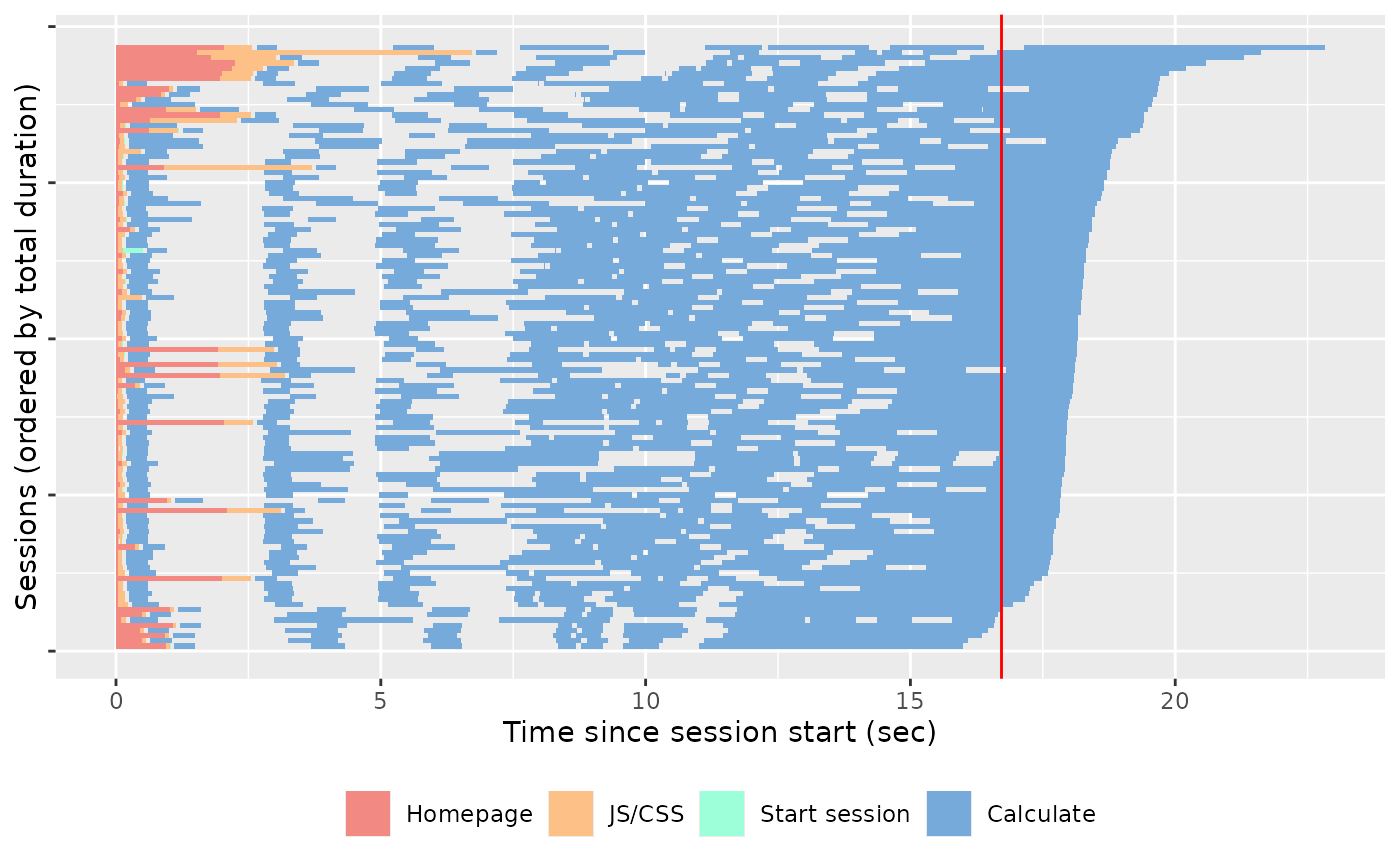slt_waterfall(slt_demo_data_4)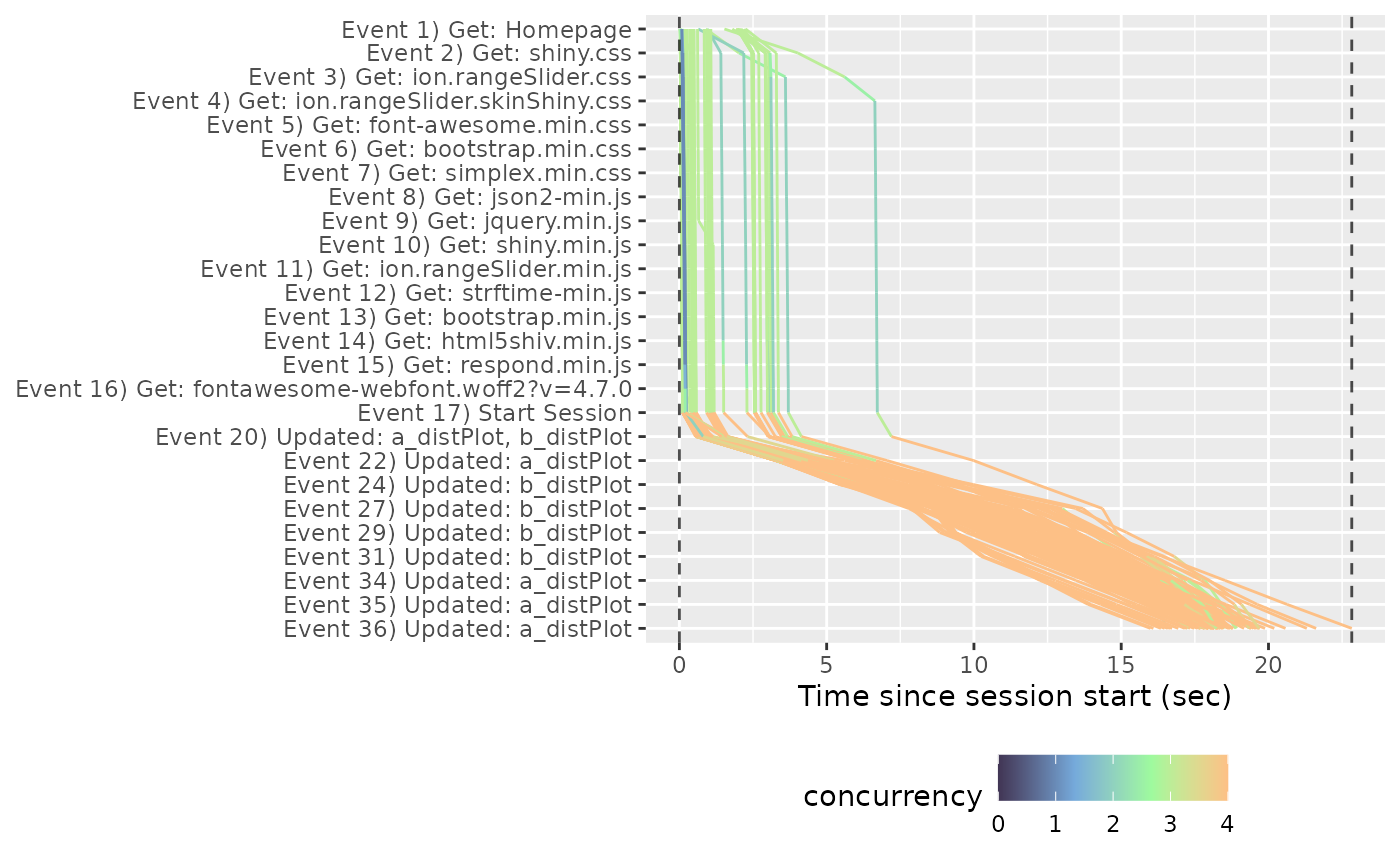slt_time_boxplot(slt_demo_data_4)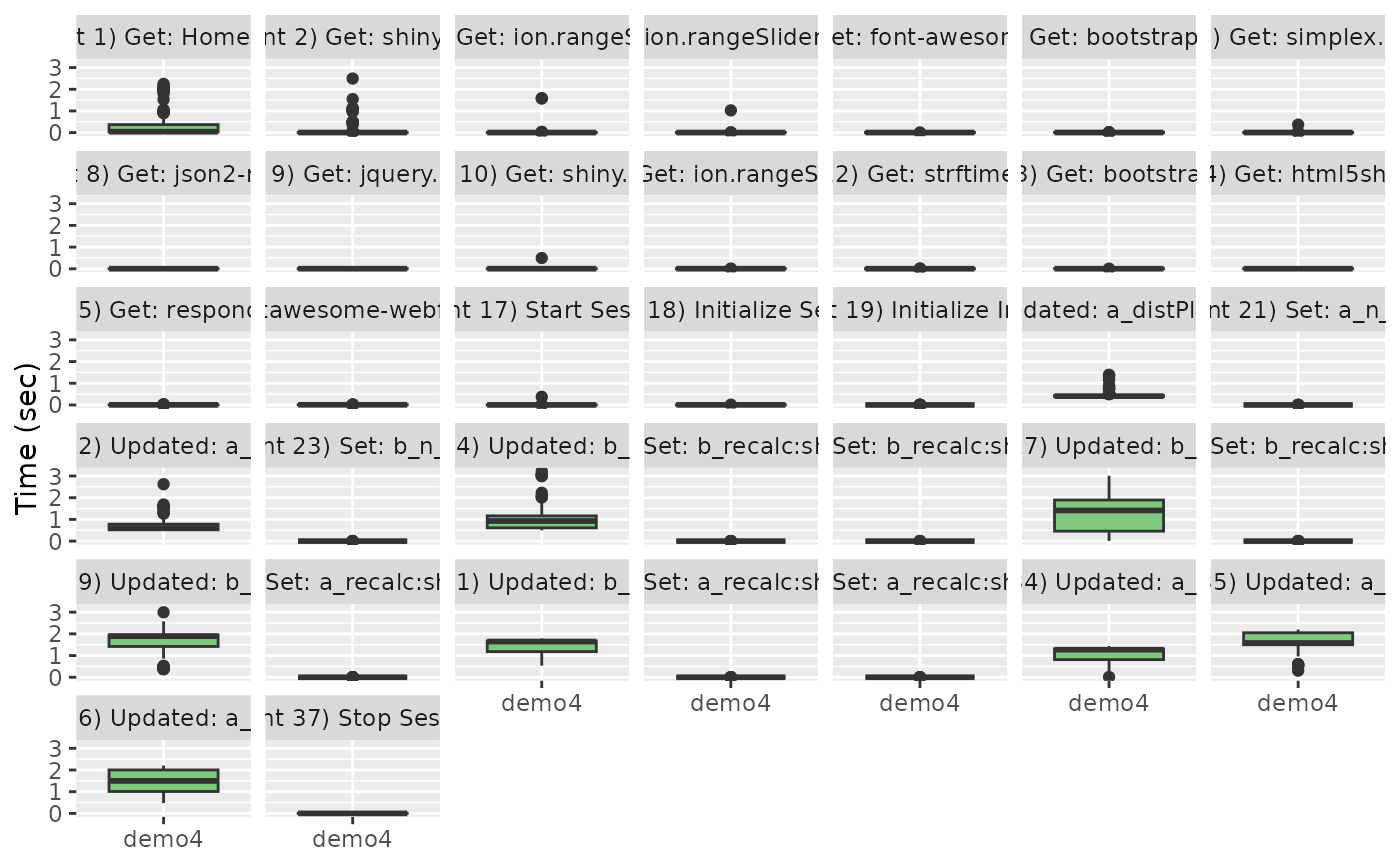slt_time_concurrency(slt_demo_data_4)slt_session_latency(slt_demo_data_4)slt_http_latency(slt_demo_data_4)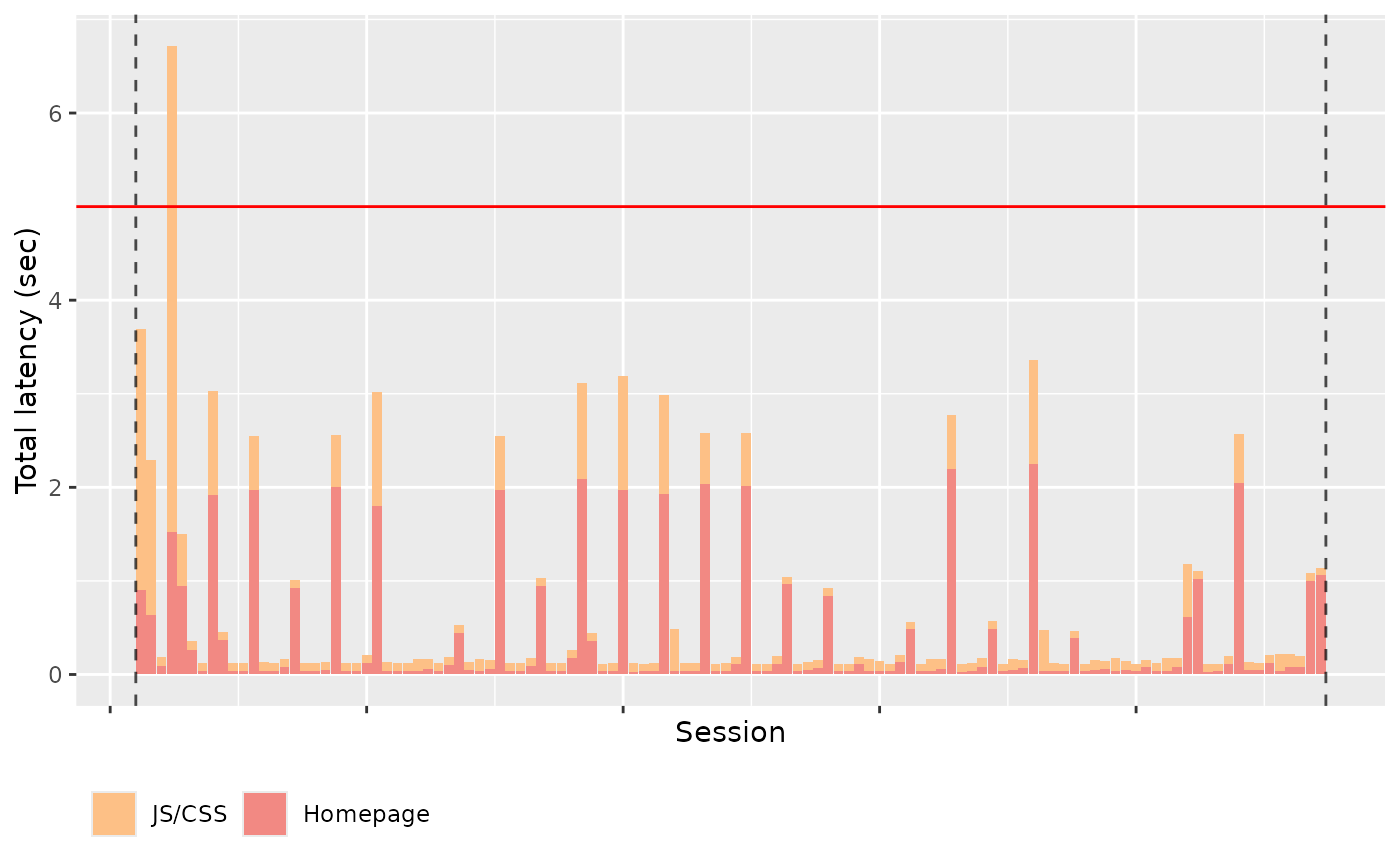slt_websocket_latency(slt_demo_data_4)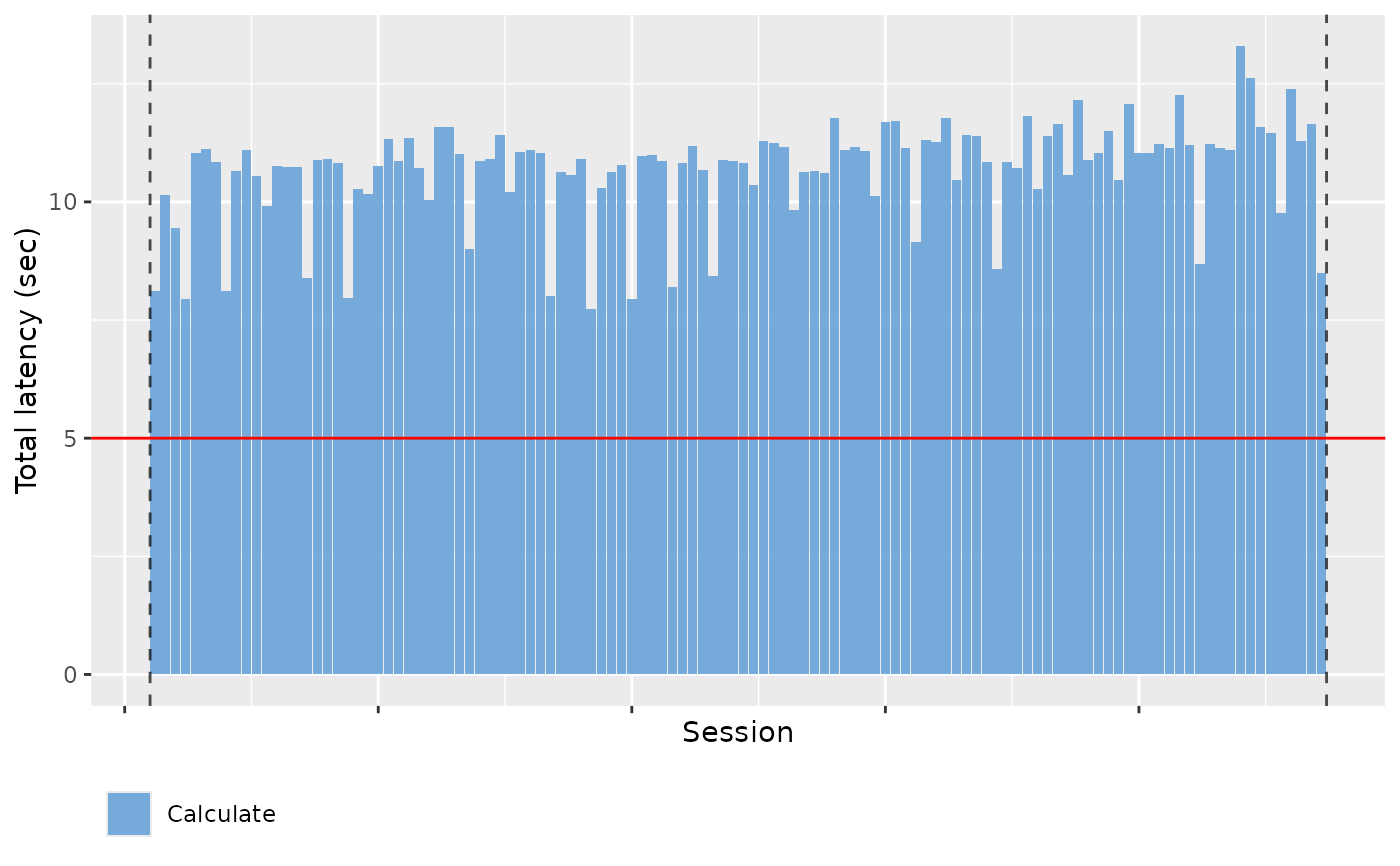#> stat_bin() using bins = 30. Pick better value with binwidth.